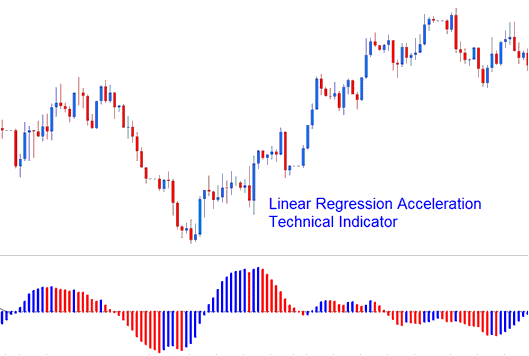# Linear Regression Acceleration Indices Technical Analysis and Indices Trading Signals

Linear Regression Acceleration calculates the change in the regression line's gradient on the current stock indexes price bar from its gradient from the previous stock indexes price bar. The value used to calculate the linear regression is referred to as the normalized acceleration value which is drawn for each stock indexes price bar formed on the stock indexes price chart.Linear Regression Acceleration

If the normalized acceleration is 0.30, then the regression line normalized slope will be rising at the rate of 0.30 per stock indexes price bar.

Similarly, a normalized slope of -0.40 would indicate that the regression line normalized slope is declining at the rate of -0.40 per stock indexes price bar.

For example, if the current stock indexes price bar normalized slope value is 0.40 and the previous stock indexes price bar normalized slope value is 0.20, then the normalized acceleration of the current stock indexes price bar would be calculated as 0.40 - 0.20 = 0.20.

NB: It is important to realize that a positive acceleration value doesn’t equate to a positive slope value, it simply means the gradient of the slope is increasing. A negative acceleration value doesn’t equate to a negative slope value, it simply means the gradient of the slope is decreasing.

## Implementation of Linear Acceleration Regression

The Linear Regression Acceleration indicator allows for the following; stock indexes price selection, regression periods, smoothing of raw stock indexes price before applying the regression and selection of the smoothing type.

The resulting regression slope is displayed as a bi-colored histogram that oscillates above & below 0.

The reference line is set at the 0 level mark.

• A rising slope; (greater than its previous value 1 bar before) is displayed in the up slope color.

• A declining slope; (lower than its previous value 1 bar before) is displayed using the down slope color.